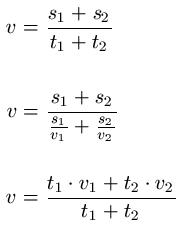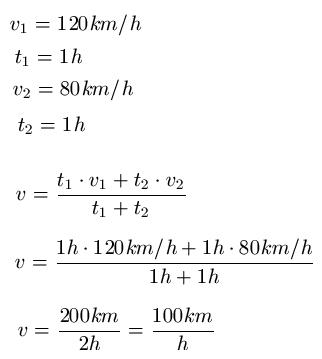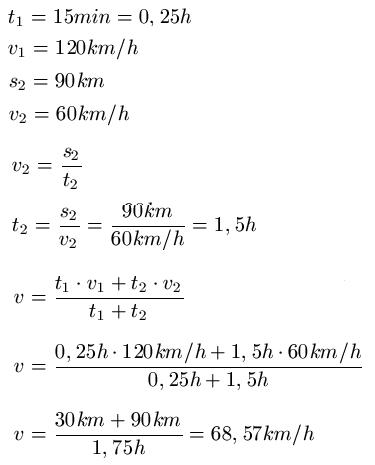# What is the formula for the average speed

## Calculate average speed

We will deal with average speed in this physics article. You will learn the formula for calculating the average speed and two examples will be presented. This article belongs to our mechanics division.Two different types of movement are often presented in physics classes. The uniform movement in which the speed is always the same and the constantly accelerated movement in which the acceleration is always the same. These are two relatively simple cases, but they only occur to a limited extent in road traffic. Because who can always drive the same fast? For this reason, in this article, we care about the average speed. By the way: If you still have problems with the uniform movement or the accelerated movement, you can read about them in the following two articles:

Show:

### Average speed: definition and formula

The average speed is the mean of all the speeds that were driven on a specific route in a specific time. For example, a car could travel at a speed of 120 km / h for one hour on the motorway and then a speed of 80 km / h for another hour on the country road. We'll deal with such examples in the same way. The following formulas can be used to calculate the average speed:

Average speed formulaWhere:

• "v" the average speed in meters per second [m / s]
• "v1" is the speed for the first stretch in meters per second [m / s]
• "v2" is the speed for the second distance in meters per second [m / s]
• "s1"is the first distance in meters [m]
• "s2"is the second distance in meters [m]
• "t1"is the time for the first distance in seconds [s]
• "t2"is the time for the second route in seconds [s]
Show:

### Examples of the average speed

As a rule, one understands content much better through examples. Hopefully, the following average speed word problems will help you out.

Example 1:

A car on the motorway drives at a speed of 120 km / h for one hour and then on the country road at a speed of 80 km / h for another hour. What is the average speed?

Solution: We take all the information from the text and insert it into the formula for calculating the average speed.Example 2:

A woman drives at a constant 120 km / h for 15 minutes. However, she can only drive another 90 km at 60 km / h per hour. Calculate the average speed.

Solution: First we take all the information from the text. Unfortunately, we cannot insert this directly into a formula because not all of the information is available. For this reason we first calculate the second time. And then we put all the information in the corresponding formula.Left:

### Who's Online

We have 236 guests online